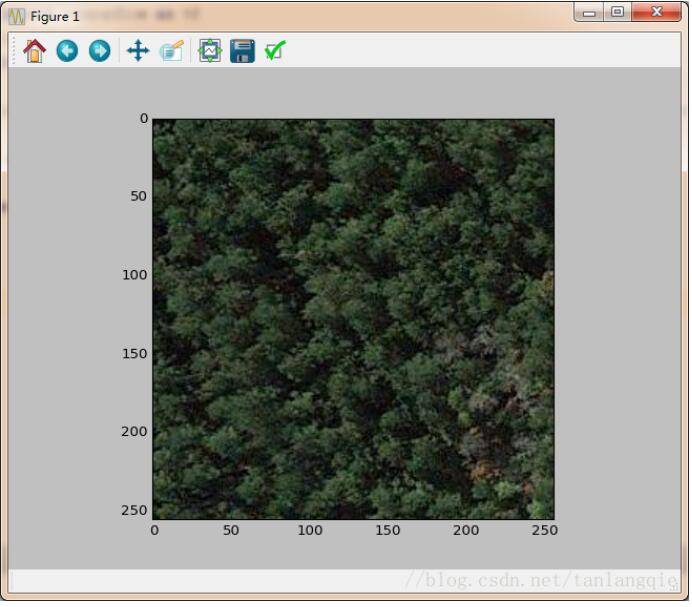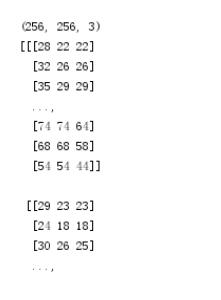# python读取图片的方式,以及将图片以三维数组的形式输出方法

``````
import numpy as np
from PIL import Image
import matplotlib.pyplot as plt

plt.imshow(image)
plt.show() #将图片输出到屏幕

image_arr = np.array(image) #将图片以数组的形式读入变量
print (image_arr)
``````

``````
# coding=utf-8
import tensorflow as tf
import numpy as np
import matplotlib.pyplot as plt

image = tf.image.decode_jpeg(image_contents, channels=3) #解码jpeg

with tf.Session() as sess:
sess.run(tf.global_variables_initializer())

img=sess.run((image)) #img为三维数组
print (img.shape) #输出数组形状
print (img) #打印数组

plt.imshow(img) #显示数组
plt.show()
``````python 读取 图片 三维数组 输出